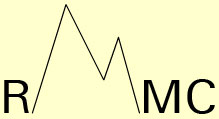Journal of
Integral
Equations
and
Applications

Editorial Board

Information for Authors

Abstracts
and
Complete text

DOI 1988-2007

Subscription Information

Accepted Papers

RMMC# Journal of Integral Equations and Applications

The Journal of Integral Equations and Applications is an international journal devoted to research in the general area of integral equations, fractional differential equations and their applications.

The Journal of Integral Equations and Applications, founded in 1988, endeavors to publish significant research papers and substantial expository/survey papers in theory, numerical analysis, and applications of various areas of integral equations, and to influence and shape developments in this field.

The Editors aim at maintaining a balanced coverage between theory and applications, between existence theory and constructive approximation, and between topological/operator-theoretic methods and classical methods in all types of integral equations. The journal is expected to be an excellent source of current information in this area for mathematicians, numerical analysts, engineers, physicists, biologists and other users of integral equations in the applied mathematical sciences.

Science Citation Index.Journal of Integral Equations and Applications has been accepted for the following Thomson Reuters products:
Science Citation Index-Expanded (SCIE) including the Web of Science